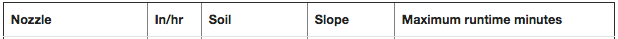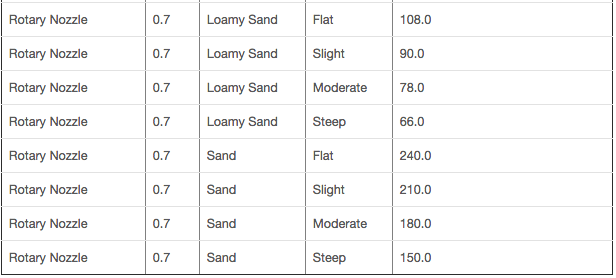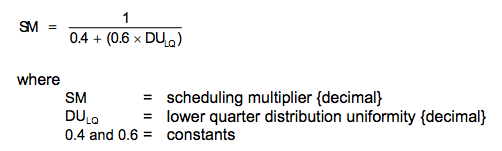Smart Cycle Question

@derek_USSI, as @plainsane asked, how did you calculate this value? If your efficiency (DU) is 80%, you’ll need to run the sprinklers longer to achieve a net PR of .39 in/hour.

The Landscape Irrigation Auditor Handbook (3rd Edition) provides the following equation for determining your watering times:

RT = D/PR * 60

• RT: run time (minutes), lower boundary
• D: depth of water to apply (inches)
• PR: Precip Rate (in/hr)

Taking the lower boundary RT, DU is used to calculate the upper boundary RT, which is simply multiplied by the scheduling multiplier – which is a fixed valve based on the DU %.

Assuming DU is 80%, the scheduling multiplier would be 1.14.

Example:

• RT: unknown
• D: .50 inches
• PR: .39 in/hr

RT (lower) = 76.9 = 77 minutes

RT (upper) = 77 * 1.14 = 87.78 = 88 minutes

The Irrigation manager should select a run time between the upper and lower boundaries; the Iro takes the average of the two.

From here, the RT would be cross referenced to the Smart Cycle table as outlined in this support article. Please note, these are maximum allowable watering minutes as outlined by the EPA for WaterSense testing.

I hope this helps. In short, Smart Cycle works by taking the RT (either Rachio calculated, or user set) and is cross referenced to the Smart Cycle table. The number of cycles is determined by the maximum number of cycles needed amongst the zones in the watering schedule; i.e. if one zone needs 2 cycles and another needs 4 cycles, all zones will be cycled 4 times.

@plainsane, good point. The only way to calculate the true precip rate is to conduct a catch cup test.

1 Like

@emil
@plainsane
I don’t have to derive it. It’s straight from the MP Rotator nozzle chart. https://www.hunterindustries.com/node/501

And this is most likely what one would use when customizing a nozzle.
That said you can derive it by the following formula:

PR (gross) = (96.25 x gpm) / (H x R)

gpm = flow of full circle sprinkler
H = head spacing
R = row spacing

And yes, not from one nozzle, but from one type of nozzle that all have a matched precipitation, weather by nozzle design( Rainbird MPR’s) or by choosing the appropriate nozzle output (rotors). But this only gives you the water leaving the nozzle, PR(gross). And yes this would be a higher amount of water as this would assume all water leaving the nozzle gets to where it is suppose to. The water hitting the ground would be this number multiplied by your distribution uniformity (DU) giving you your PR(net). And yes you can find your DU and your PR(net) by attempting a catch can test.
But how do you do a catch can test for a shrub zone?
And how accurate would they be if like in the video the homeowner walks out with the 9 catch cans, to his 1/4 acre back yard grass?

I may not have been clear. You said that “It’s important to remember that drip nozzles (emitters, bubblers, & misters) do not use Smart Cycle since their PR is usually below IR” but if one creates a custom nozzle of an MP Rotator, which has a PR of 0.39 in/hr (below the IR of most soils) the software will not allow it to Smart Cycle the same as drip nozzles aren’t allowed?

Well, correct me if I’m wrong, but I though to get gpm you sum the gpm from all the heads?
This is an assumption I have made following I’m just following this guide here, http://www.hunterindustries.com/sites/default/files/design_guide_MP_Rotator_LIT-461_dom.pdf

So if you had the head to head coverage as they recommend, I’m convincing myself that the pr is higher than what the manual lists for pr as you can have multiple heads watering the same square foot of patch. Am i wrong on this understanding?

I think the spirit of the video is to keep it simple

@derek_USSI, sorry I wasn’t clear in my question – I was referring to the .3 with a DU of 80%. This should not be multiplied directly against the precipitation rate as the app does this for you using the Irrigation Association’s scheduling multiplier formuala (SM=(1/(0.4+0.6×DUlq)) accessible in the advanced settings for each zone.

Usually drip zones can be calculated using the emitter output on a per shrub basis. If the emitters and/or vegetation type vary, scheduling via the app is difficult as root zone depth and precip rates would vary. Out of curiosity, what type of nozzle is being used in this zone?

We might need to find a different instructional video for larger lawns– its intended use was to serve as a guide to help explain the concept of what a catch cup test is.

This is correct regarding drip nozzles; however a MP Rotator would classify as a rotary nozzle:

Your PR(gross) would be the same as you can’t change the water coming out from the nozzle. And if all the nozzles in the zone put out the same amount of water for the area they are to cover (eg with rotors: corners = 1 gpm, halves = 2 gpm, fulls = 4 gpm). Your PR(net) would change because if you had a well designed system with good head to head coverage them you distribution uniformity would be closer to 100% which would mean more water hitting the ground where you want it. PR(net) = PR(gross) x DU. Poor design and mixed nozzle outputs will affect your DU calculation when you do a catch can test and, therefore, affect your PR(net).
So in the example above, if these rotors shot 25 ft by the formula you would have a PR(gross), water from the nozzles of 0.62 in/hr. That said if you did a catch can test and calculated a DU of 80% (or left thwe Iro to its own as the preset is 80%) the water hitting the ground would be 0.49 in/hr. which is your PR(net). This is then the amount of water used to calculate a run time as this is the water hitting the ground.
Therefore, you can understand, if you have a low flow nozzle like the MP Rotator you could end up with long run times depending on your DU and the root depth.
Trust me, I’m trying to understand this, so if I’m wrong please let me know.

You and me both brotha man, after some more reading last night, these mp rotators seem like a hardware solution to smart cycling, and I recommend turning it off on the iro for those zones. If I understand there documentation…smart cycling is redundant in this case. Then all you need to understand is how the flex scheduler works, which will inform the homeowner, how rachio determines the next water application.

@derek_USSI, correct. Going back to Robert D. von Bernuth’s feedback, …the run time until runoff increases without bound as the PR approaches the IR…when PR is equal to or less than IR, you can run indefinitely.

@plainsane, yea that was the goal for these nozzles since most timers don’t have cycle/soak capability. Per Hunter’s website, MP Rotator nozzles feature unique, multi-trajectory rotating streams that deliver water at a steady rate. This slower application rate allows water to gently soak in at rates that soils can absorb.

@emil
True. And as Franz said above they wouldn’t cycle. But what happens when you set them in a Flex schedule?

If in a Flex schedule you are letting the tank go empty (50% for plants) then you could get some very long run times because it would be like filling the tank with a straw!
Right?

@derek_USSI, yes that is correct (and a good analogy). When using a rotary nozzle, Smart Cycle would kick in if any of the zone durations exceed the following based on the zone attributes selected:NOTE: This assumes a nozzle precipitation rate of .7 in/hour & using the default available water settings for the soil type selected

1 Like

Yes, but this isn’t the max time to fill the tank. This is the max time before cycling occurs, which could increase the total amount of time to water a zone because there is an amount of time in between cycles (30 min?) that it needs to rest. Right?

And your Run Times before Cycling are based on a 100% DU, right?

And this would be the extension based on a client creating a Custom MP Rotator nozzle? Would this be correct? And at a DU of 80% the PR(net) is less than the IR so making is not cycle at all.

@derek_USSI, the water duration is fixed per the schedule settings. These can be edited to your preference. To do so on a Fixed schedule, see #3 in this support article. To do so on a Flex schedule, use the water droplet icon as detailed in this support article.

The total duration of the schedule might increase if Smart Cycle is enabled, but the actual watering duration will not increase.

DU would increase your total watering pending the percentage; it doesn’t impact the max runtime mins. It would simply require additional cycles to complete the total watering duration.

In the data table you posted, could you provide some insights into the math you’re doing?

A 80% DU would result in a longer watering duration. If it exceeds the max runtime, then Smart Cycle would kick in. As a remember, this is how the Iro uses DU:

Assuming DU is 80%, the scheduling multiplier would be 1.136 = 1.14.Using DU to calculate the watering time:

• RT: unknown
• D: .50 inches
• PR: .39 in/hr

RT (lower) = 76.9 = 77 minutes

RT (upper) = 77 * 1.14 = 87.78 = 88 minutes

What Is Smart Cycle
The Maximum Runtime allowable before runoff occurs will be calculated from the following formula:
Rt (max) = 60 (ASA)/(PR – IR), minutes
ASA = Allowable Surface Accumulation
PR = Precipitation Rate. The amount of irrigation water applied per unit of time.
IR = Soil intake rate

So using your above formula,

How is D:depth of water to apply, derived? Its the ETo x Crop Coefficient, right?

It’s becoming clearer. If you don’t use/adjust the PR in this formula using the DU you wouldn’t get below 0.39 and as this is the lowest output nozzle I can find you may not have infinite cycle times.

@derek_USSI, great question. Depth of water is derived from the vegetation selection (which tells us the root zone depth in inches) & soil selection (which tells us the available water per inch). Multiplying these two values provides us with the plant’s available water (PAW), or how big of a glass the plant needs. Allowed depletion (which tells us how much of the “glass” we need to refill) is simply a percentage of PAW. An example would be:

Vegetation: Cool season grass (6 inches)
Soil: Loam (0.17 in/in)
PAW = 1.02 inches

Allowed Depletion: 50%
Irrigation Amount = .51 inches.

Then, using the precip rate of the nozzle and ETo X Crop Coefficient, we can build out the scheduling requirements of the zone.

Yea, think of PR & DU as two independent variables that can change.

In the example above, a PR of .39 w/100% DU = RT (lower) = 1.31 hours = 79 minutes. However, if the DU was 80%, all else constant, RT (upper) = 79 * 1.14 = 90 minutes.

The Maximum Runtime allowable via Smart Cycle would be:
Rt (max) = 60 (ASA)/(PR – IR), minutes

• ASA: .3 (no slope, loam soil)
• PR: .39 in/hr
• IR: .35 in/hr

Rt = 60 (.3)/(.39-.35) = 18/.04 = 450 minutes. Assuming PR was less than IR, Smart Cycle would never need to kick in as you’d be watering less than the infiltration rate of the soil.

Let me know if this helps. If not, maybe we could schedule some time for a phone call to go into the details further. I appreciate your questions related to this subject and I want to make the math and science easier for everyone to understand.

Best, Emil

1 Like

I realize this has taken a lot of your time and I am very thankful. It is really about the customer, or in my case the technician, understanding enough so that when odd things happen or we get questions like Flex schedule with long intervals we can realize how things work and manipulate or personalize things to make the system work better. I believe @franz said it best somewhere, “garbage in, garbage out.” And a 0.7 in/hr output is quite different than a 0.39. And root depth in Arizona might be quite different than Seattle. People, and technicians, need to realize that the preset values are guidelines. I believe you have a great product and even better customer support, but my clients are not going to log into this community. Some of my client do not even know where there timer is. That’s why it’s important to not only support those who personally take interest in their irrigation system but also those companies who, believing this is a good product, install them into their customers homes and have to deal with these questions themselves.
Again, thank-you for all the input. You really have been fantastic.

2 Likes

@derek_USSI, happy to help and explain. You’ve asked great questions and I couldn’t agree more with your statements. We’re working on improving our educational resources available for programming the Iro and detailing what & how all values/inputs work. As you’ve seen, there are numerous data points that if incorrect, will result in poor decisions by the Iro.

Our of curiosity, how do you think we could better educate technicians? What initially caught your eye about Rachio?

Thanks again for your support. Keep asking questionsBest, Emil

1 Like

Good question, Emil! I will give it some thought over the Winter.

1 Like